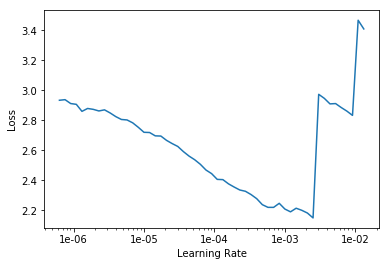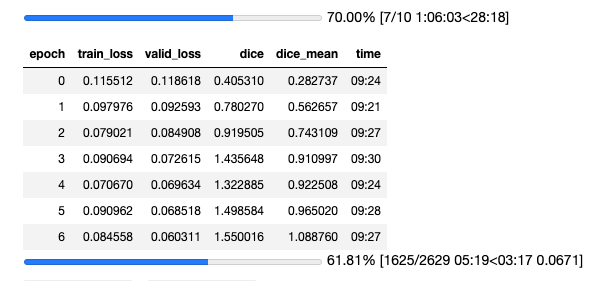# Training UNET for segmentation negative dice score

I am training a UNET for segmentation (10 classes) at my work place. Input image size is 4250 * 5500 , which is resized to 960 * 720 ,so that its fits into memory.

I have only 332 input images , which i am splitting into training 243 records and validation 89 records.

Am seeing negative dice score. Is that because i do not have sufficient data ?. Could you please suggest what i can do to make the dice score better.

1 Like

Could you provide the code you are using? Without it is hard to help.

I doubt data volume is a big issue. I’ve had fortune with just one 4000x6000 image - split into 256x256 tiles - let alone 30.
I think you either need to show some code or input/truth/press images to help more.

1 Like

thank you . I am using lesson3-camvid.ipynb as the base code. Loss function is the default FlattenedLoss of CrossEntropyLoss() of the unet_learner. Dice as the metric.

Have 243 records in the training set and 89 records in the validation set.

Started with a small size of 360, 480 and bs = 4.

Learning rate finder as belowlr= 1e-5
learn.fit_one_cycle for 12 epochs

epoch train_loss valid_loss dice time
0 2.607163 2.373696 -1.597472 00:36
1 2.136024 1.833057 -1.627554 00:33
2 1.726768 1.329626 -1.623146 00:33
3 1.450157 1.162594 -1.615568 00:33
4 1.288652 1.076266 -1.591018 00:33
5 1.196529 1.035175 -1.578016 00:33
6 1.087081 1.084165 -1.620262 00:33
7 1.096788 1.023510 -1.590258 00:33
8 1.016304 0.992499 -1.601955 00:33
9 0.984961 0.976767 -1.617078 00:33
10 0.978132 0.977125 -1.579850 00:33
11 0.942967 0.999436 -1.577580 00:33

What concerns me is the negative dice score ? . May be its because i do not have sufficient data.

Do you use an adapted dice score that computes a dice score per class? I think the problem could be the use of 10 classes. Maybe you should try to take the model after 12 epochs and generated predictions. Then, compute the dice score on its own for each class, which can then be averaged. Either you spot an error in the function by doing so, or you find a flaw in your usage of dice score. Hope that helps.

2 Likes

thank you

I was checking the dice function and noticed that when taking the dice of a mask with itself, I’m not getting the expected value of 1.0. Am I missing something?

Hi,

Ia m training a Unet learner and using dice (built-in metric) co-efficient and dice_mean (as shown below). However I am getting the metric score more than 1. I am not sure the reason behind this. Is this as expected or am I doing something wrong.

dice mean:

def dice_mean(input, target):
“Dice coefficient metric for binary target.”

``````# Threshold targs
n = target.shape
input = input.argmax(dim=1).view(n,-1)
target = target.view(n,-1)

# Compute dice
intersect = (input * target).sum(dim=1).float()
union = (input + target).sum(dim=1).float()
dice = 2. * intersect / union

# Replace zero union values with 1
dice[union == 0.] = 1

# Return mean
return dice.mean()
``````I am getting the same, did you find a solution to this?

I was also facing the same issue, after digging for a while came to know that the built-in dice metric was not correct for segmentation with more than 2 classes. I think it was built for binary classification of pixels and that to classes being 0 and 1. So I made a new dice metric by changing the existing one.

Dice coefficient for multi_class_segmentation

def dice_multi(input:Tensor, targs:Tensor, iou:bool=False, eps:float=1e-8)->Rank0Tensor:
n = targs.shape
targs = targs.squeeze(1)
input = input.argmax(dim=1).view(n,-1)
targs = targs.view(n,-1)
targs1 = (targs>0).float()
input1 = (input>0).float()
ss = (input == targs).float()
intersect = (ss * targs1).sum(dim=1).float()
union = (input1+targs1).sum(dim=1).float()
if not iou: l = 2. * intersect / union
else: l = intersect / (union-intersect+eps)
l[union == 0.] = 1.
return l.mean()
Thanks.